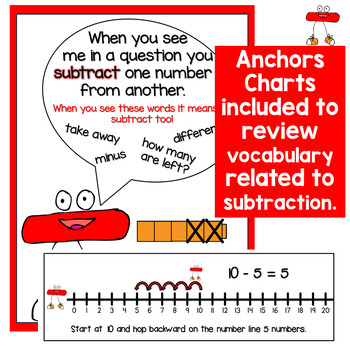DID YOU KNOW:
Seamlessly assign resources as digital activities

Learn how in 5 minutes with a tutorial resource. Try it Now

Learn More# Math Craft for Subtraction | Linus the MinusK - 2nd
Subjects
Standards
Resource Type
Formats Included
• PDF
Pages
11 pages

#### Also included in

1. Teaching Addition and Subtraction? Your students will love making these math craftivities - Gus the Plus and Linus the MInus. Also included in this pack are personal number lines, anchor charts and printables and worksheets. Included in this resource are the following:⭐Gus the Plus Addition Crafti
\$4.50
\$5.00
Save \$0.50

### Description

Introduce your students to subtraction with this math craftivity for subtraction - Linus the Minus. This pack includes the Linus the Minus Craft, a ready to use anchor chart, a subtraction card to add to your math word wall, personal number lines for students and two worksheets to practice subtraction with a number line.

Save money and purchase this as part of a money saving BUNDLE

Addition & Subtraction Craftivity - Gus the Plus and Linus the Minus

Included in this resource are the following:

⭐A Linus the Minus Subtraction Craftivity.

⭐An Anchor Chart for Subtraction.

⭐A card explaining Subtraction for your math word wall.

⭐Number lines for students to use for independent practice.

⭐2 worksheets for subtraction.

How to use this package:

⭐Add the Math Craftivities to your Anchor Chart with addition/subtraction words, and it is an instant visual reminder for students.

⭐Have your student create their own craftivity and have them fill out the speech bubble with their understanding of the operation.

⭐Use the included number lines with the worksheets for independent practice.

Check out some of my other Gus the Plus products:

Addition Centers with Gus the Plus

Addition to 20 Clip Cards with Gus the Plus

Read and Write the Room - Addition to 10 with Gus the Plus

Gus the Plus and Linus the Minus Fact Families to 20

Gus the Plus Addition to 10 BUNDLE

--------------------------------------------------------------------------------------------------------

Don't Miss Out:

Leave feedback on this item to earn credit toward future TpT purchases! Be the first to know about my new products and freebies! Look for the green star near my store name and click it to become a follower. You will receive customized e-mail updates about my store!

Connect with me via social media and my blog to see how I use my products in my own classroom!

Hanging Around in Primary on Facebook

Hanging Around In Primary Blog

Total Pages
11 pages
N/A
Teaching Duration
N/A
Report this Resource to TpT
Reported resources will be reviewed by our team. Report this resource to let us know if this resource violates TpT’s content guidelines.

### Standards

to see state-specific standards (only available in the US).
Add and subtract within 20, demonstrating fluency for addition and subtraction within 10. Use strategies such as counting on; making ten (e.g., 8 + 6 = 8 + 2 + 4 = 10 + 4 = 14); decomposing a number leading to a ten (e.g., 13 - 4 = 13 - 3 - 1 = 10 - 1 = 9); using the relationship between addition and subtraction (e.g., knowing that 8 + 4 = 12, one knows 12 - 8 = 4); and creating equivalent but easier or known sums (e.g., adding 6 + 7 by creating the known equivalent 6 + 6 + 1 = 12 + 1 = 13).
Use addition and subtraction within 20 to solve word problems involving situations of adding to, taking from, putting together, taking apart, and comparing, with unknowns in all positions, e.g., by using objects, drawings, and equations with a symbol for the unknown number to represent the problem.
Decompose numbers less than or equal to 10 into pairs in more than one way, e.g., by using objects or drawings, and record each decomposition by a drawing or equation (e.g., 5 = 2 + 3 and 5 = 4 + 1).
Solve addition and subtraction word problems, and add and subtract within 10, e.g., by using objects or drawings to represent the problem.
Represent addition and subtraction with objects, fingers, mental images, drawings, sounds (e.g., claps), acting out situations, verbal explanations, expressions, or equations.